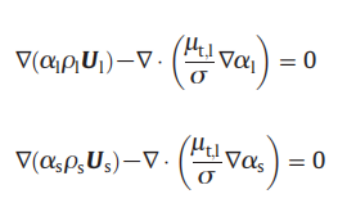# reactingtwophaseeulerfoam?

• http://dyfluid.com/reactingTwoPhaseEulerFoam.html

In the website above, equation(24) is the sum of two continuity equations. Thus, I think the source term should be added here? Please give me some suggestions.

pEqnComp1 =
(
contErr1
- fvc::Sp(fvc::ddt(alpha1) + fvc::div(alphaPhi1), rho1)
)/rho1
+ (alpha1*psi1/rho1)*correction(fvm::ddt(p_rgh))

+ fvc::laplacian(phase2.turbulence().nut()/mysigma,alpha1);


The last line is added by myself, also for pEqnComp1. I don't know why it can be run with minus that bold term while I changed the sign to be plus the simulation crashed.

• Seems you are trying to add turbulent dispersion term in the alpha equation. It is a laplacian term. A positive laplacian term usually introduce problem since it can be seen as a negative negative laplacian term, which behaves as anti-diffusion. You can follow your step to construct pressure equation including the turbulent dispersion force as a source term. Another method is to employ to operator splitting method that you can simply solve the turbulent dispersion as a last step. In this manner the pressure equation is retained.

• t is a laplacian term. A positive laplacian term u

Thanks a lot, dongyue. I check the term I implemented are both the positive and negative values. Why minus has no problem and plus occurs the problem. Sorry, what do you mean follow my step to introduce a dispersion force as source term? and Another operator is?How to split ? One thing needs to be clear, here I did not use Sp or SuSp, but do you think it will lead different results?

• @东岳 !This is the mass conservation equation I am using. The sign of laplacian term here is minus. However, as your page http://dyfluid.com/reactingTwoPhaseEulerFoam.html in equation(24), it should be plus I think if I understand well. I am confused that the sign of source term will induce large different result or no?

• The source term in Eq. (24) does not come from the laplacian term. It comes from the convection term. Eq. (24) is not related to your posted equations. The laplacian terms in your posted image are turbulent dispersion terms. In OpenFOAM they are not implemented in this manner.

• @东岳 If I understand well, equation (24) was changed by the sum of two mass conservation equation. However I must add that term in the mass conservation equation. So the position I implemented the laplacian term is right? The mass conservation equation shown in picture is our own equation.

• If you sum them up, $-\nabla\cdot(\nabla\alpha_l+\nabla\alpha_s)=0$ This term disappears.

• @东岳 Thanks, if the sum of that two term is zero, so there cannot exist any problem during the simulation. why I use the sign "plus", it crashed while 'minus' run well? And if I changed the phase2.turbulence.nut() to be nu() a constant, "plus" has no problem?

• why I use the sign "plus", it crashed while 'minus' run well? And if I changed the phase2.turbulence.nut() to be nu() a constant, "plus" has no problem?

Do you obtain physically reasonable results? or just not blowing up.

• @东岳 Frankly, I use the minus sign and I got the results however they doesn't agree well with the results from PHOENICS. The velocity profile seems shift in some degree. So I would like to try "plus" this term. I am not sure the place I added is right or not. When I change one to be plus and the other to be minus, it runs well and the results seems better.

• You cannot implement equations as you want. What you implement should obey physics. Otherwise even you get "reasonable" results, its just coincidence.

• @东岳 I see. That is the reason why I post here. I wanna be sure that term should be implemented in which place. This is what I expect. Do you have any idea?

• @东岳 I am using IPSA model (lauder and spalding). In all of equation used, the alpha term has been introduced inside.
http://www.cham.co.uk/phoenics/d_polis/d_lecs/ipsa/ipsa.htm#11

• @东岳 Sorry. I am still confused that how the equation(24) mentioned in your page could be solved in OpenFoam. In the source code:

        solve
(
pEqnComp1() + pEqnComp2() + pEqnIncomp,
mesh.solver(p_rgh.select(pimple.finalInnerIter()))
);


why pEqnComp1() + pEqnComp2() + pEqnIncomp ranther than - pEqnComp1() - pEqnComp2() + pEqnIncomp. Because in your equation(24), pEqnComp1() + pEqnComp2() in the right side while pEqnIncomp in the left side.

• Thanks for your feedback. The R.H.S. of the following equation should be a - sign. It was corrected.
\begin{equation}\label{comp_nablaU}
\underset{\mathrm{pEqnIncomp}}{\underbrace{\nabla\cdot\left(\alpha_\rd\bfU_\rd+\alpha_\rc\bfU_\rc\right)}}=\underset{\mathrm{\color{red}{-}pEqnComp1\color{red}{-}pEqnComp2}}{\underbrace{-\frac{\alpha_\rd}{\rho_\rd}\frac{\rD\rho_\rd}{\rD t}-\frac{\alpha_\rc}{\rho_\rc}\frac{\rD\rho_\rc}{\rD t} }}.
\end{equation}

What is the first term? Its a second order tensor which is not consistent with the second term (scalar).• @东岳 So if I wanna add the term related to the gradient alpha in the continuity equation, in which place should I put the term?

• @东岳 Thanks, Dongyue. I am still confused that why I add the same term in the pEqnComp1 and pEqnComp2, or pEqnIncomp leads to the different results?

• So if I wanna add the term related to the gradient alpha in the continuity equation, in which place should I put the term?

You should implement it in the alpha equation.

• @东岳 Sorry, to my knowledge alpha equation dosn't exsist in the twophaseeulerfoam? How can I realize it?

• alpha field was solved by MULES algorithm. You can add something after MULES procedure as follows:

solve(fvm::ddt(alpha) - fvm::laplacian(D, alpha) = fvc::ddt(alpha);


• @东岳Thanks. however MULES is not implemented in twophaseeulerfoam. As mentioned before, the term must be added into the continuity equation rather than alpha equa, which is the Spalding's IPSA solver in PHOENICS.

• @kimy Sorry, I mean where is the MULES in the twophaseeulerfoam, which I did not find but I found MULES in the terminal during calculation.

• alpha was solved in twoPhaseSystem.C